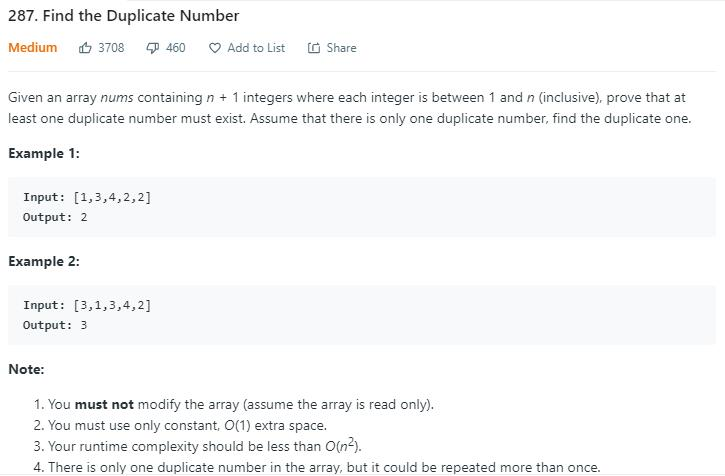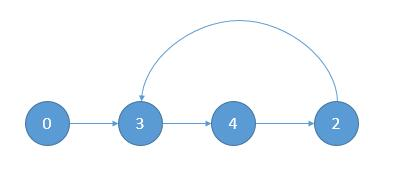# 题目描述（中等难度）1n 范围内的某些数，放到大小为 n + 1 的数组中，数组要放满，所以一定会有一个重复的数字，找出这个重复的数字。比如 [2,2,2,1]

# 解法一 排序

public int findDuplicate(int[] nums) {
Arrays.sort(nums);
for (int i = 0; i < nums.length - 1; i++) {
if (nums[i] == nums[i + 1]) {
return nums[i];
}
}
return -1;
}


# 解法二 HashSet

public int findDuplicate(int[] nums) {
HashSet<Integer> set = new HashSet<>();
for (int i = 0; i < nums.length; i++) {
if (set.contains(nums[i])) {
return nums[i];
}
}
return -1;
}


# 解法三 二分查找

mid = (1 + n) / 2，接下来判断最终答案是在 [1, mid] 中还是在 [mid + 1, n] 中。

public int findDuplicate(int[] nums) {
int n = nums.length - 1;
int low = 1;
int high = n;
while (low < high) {
int mid = (low + high) >>> 1;
int count = 0;
for (int i = 0; i < nums.length; i++) {
if (nums[i] <= mid) {
count++;
}
}
if (count > mid) {
high = mid;
} else {
low = mid + 1;
}
}
return low;
}


# 解法四 二进制

1 3 4 2 2 写成 2 进制
1 [0 0 1]
3 [0 1 1]
4 [1 0 0]
2 [0 1 0]
2 [0 1 0]

1 [0 0 1]
2 [0 1 0]
3 [0 1 1]
4 [1 0 0]

1 到 4 最后一列 1 的个数是 2
2 不大于 2,所以当前位是 0, res = XX0

1 到 4 倒数第二列 1 的个数是 2
3 大于 2,所以当前位是 1, res = X10

1 到 4 倒数第三列 1 的个数是 1
1 不大于 1,所以当前位是 0, res = 010



public int findDuplicate(int[] nums) {
int res = 0;
int n = nums.length;
//统计每一列 1 的个数
for (int i = 0; i < 32; i++) {
int a = 0;
int b = 0;
int mask = (1 << i);
for (int j = 0; j < n; j++) {
//统计原数组当前列 1 的个数
if ((nums[j] & mask) > 0) {
a++;
}
//统计 1 到 n 序列中当前列 1 的个数
if ((j & mask) > 0) {
b++;
}
}
if (a > b) {
}
}
return res;
}


# 解法五nums = 3
nums = 4
nums = 2
nums = 3


public int findDuplicate(int[] nums) {
int slow = nums;
int fast = nums[nums];
//寻找相遇点
while (slow != fast) {
slow = nums[slow];
fast = nums[nums[fast]];
}
//slow 从起点出发, fast 从相遇点出发, 一次走一步
slow = 0;
while (slow != fast) {
slow = nums[slow];
fast = nums[fast];
}
return slow;
}